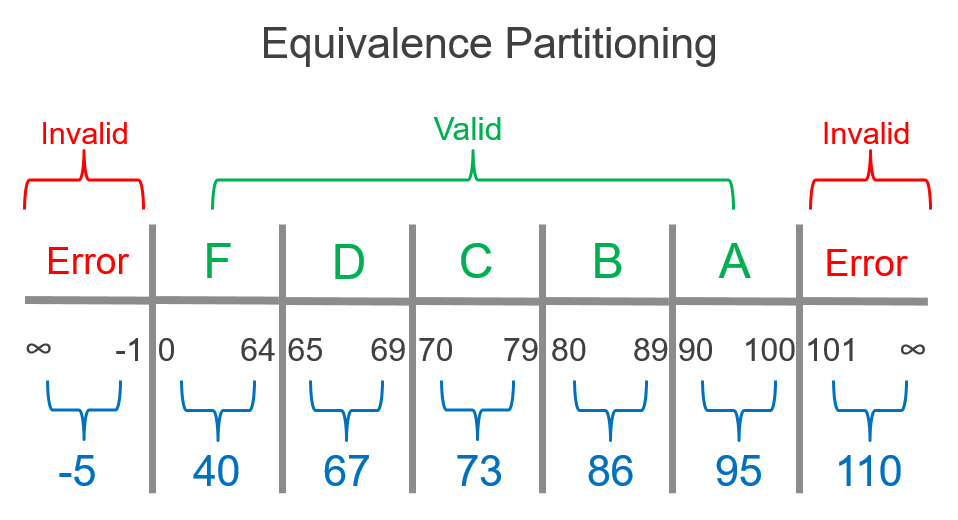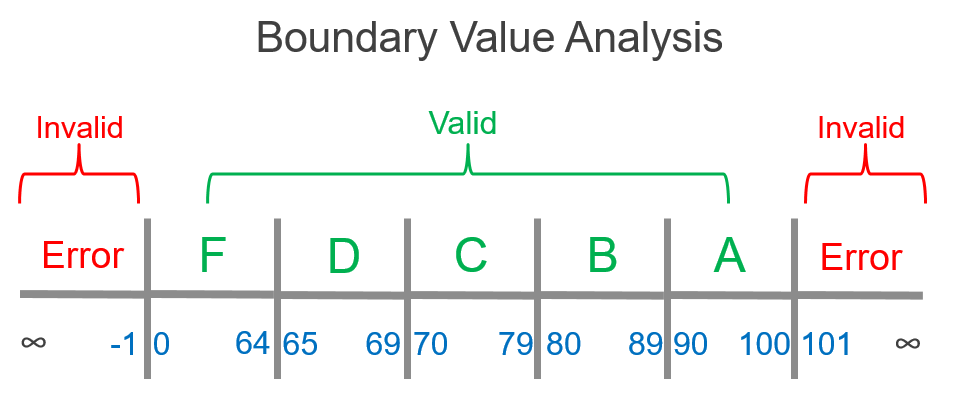# Test Design Techniques: Boundary Value Analysis

Posted in Functional Testing

Part one of the Test Design Technique series covered the importance of using equivalence partitioning.

Boundary value analysis (BVA) is a useful test design technique to use early in test design. The goal of BVA is generate test data from the boundary values of equivalence partitions. More specifically, the boundaries are the minimum and maximum values of the partitions.

Remember from part one that equivalence partitions are the groups of input data that can be considered the same. We will continue with the example used in that previous article: gradebook software used by teachers, where a student's number grade on a test is given a letter grade upon being input into the system. 0 to 64 should output F, 65 to 69 should output D, 70 to 79 should output C, 80 to 89 should output B, and 90 to 100 should output A.

The equivalence partition values came from within the range of each partition, like in this example:The boundary values are the starting and end values of each partition (of course, there is only one boundary for the open-ended partitions which extend to infinity). Boundary values are shown in blue:Given that we've already picked a value from each partition, and we assume each value in a partition is equivalent to the other values in the same partition, why should we also use the boundary values? The answer is that we will increase our chances of finding a defect if we use values from both EP and BVA. Keeping with our example, what if the range for a letter grade such as D was coded incorrectly? B consists of 80-89 and C consists of 70-79, so it would be in the realm of possibility that a programmer may have accidentally coded the D grade to include the range 60 to 69. If that were the case, our EP value of 67 wouldn't catch that. Testing the maximum boundary value of F, 64, would help find that defect.

EP and BVA use inputs that are most likely to find defects and adequately test each partition. Combining the techniques is ideal for proper test design.Partition #Input Values Expected Output
1-1, -5Error
20, 40, 64F
365, 67, 69 D
470, 73, 79 C
580, 86, 89 B
690, 95, 100A
7101, 110Error

COMING SOON: Part 3 of the Test Design Technique series will cover decision tables.